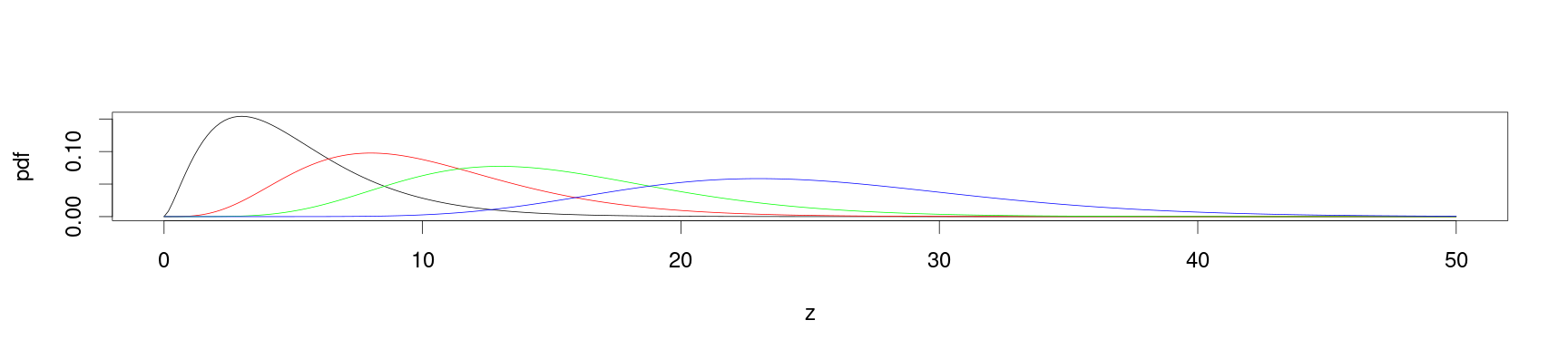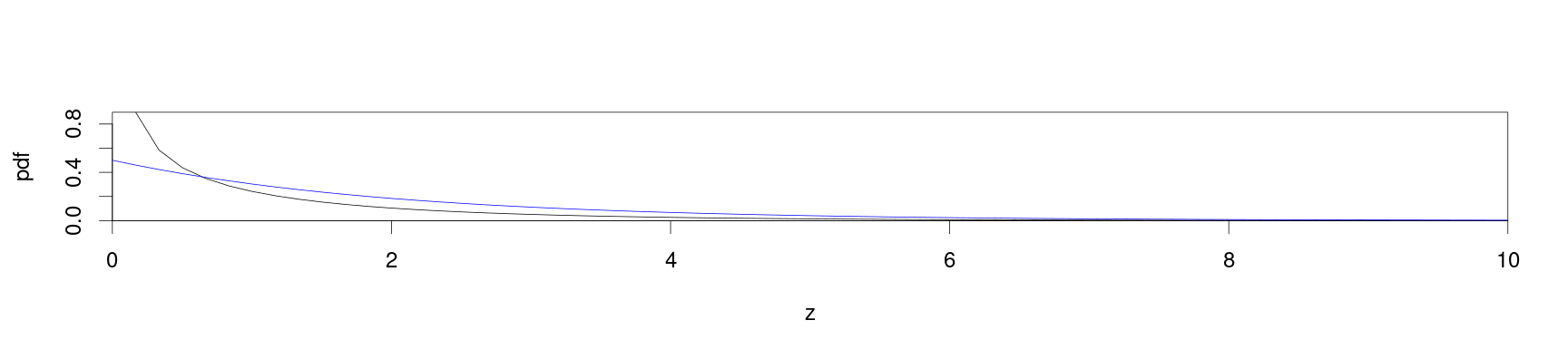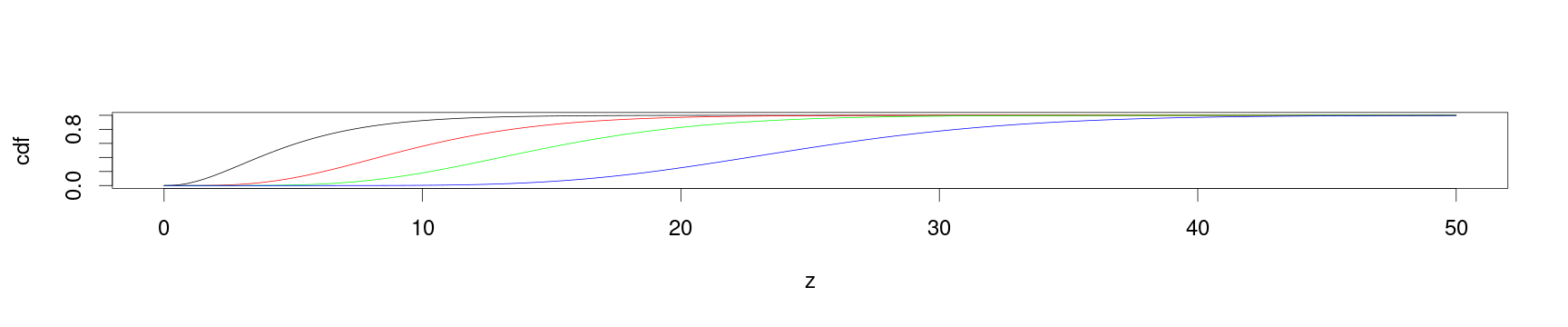# Other univariate distributions related to the normal

## Instructions:

Use the left and right arrow keys to navigate the presentation forward and backward respectively. You can also use the arrows at the bottom right of the screen to navigate with a mouse.

FAIR USE ACT DISCLAIMER:
This site is for educational purposes only. This website may contain copyrighted material, the use of which has not been specifically authorized by the copyright holders. The material is made available on this website as a way to advance teaching, and copyright-protected materials are used to the extent necessary to make this class function in a distance learning environment. The Fair Use Copyright Disclaimer is under section 107 of the Copyright Act of 1976, allowance is made for “fair use” for purposes such as criticism, comment, news reporting, teaching, scholarship, education and research.

## Outline

• The following topics will be covered in this lecture:
• The $$\chi^2$$ distribution
• The student-t distribution
• The F-distribution

## The $$\chi^2$$ distribution

• While the normal distribution is frequently applied to describe the underlying distribution of a statistical experiment, asymptotic test statistics are often based on a transformation of a (non-) normal rv.

• To get a better understanding of these tests, it will be helpful to study the $$\chi^2$$, t- and F-distributions, and their relations with the normal one.

• We will begin with the $$\chi^2$$ distribution, describing the sum of the squares of independent standard normal rvs.

• If $$Z_i \sim N(0, 1)$$, for $$i = 1, \cdots, n$$ are independent, then the rv $$X$$ given by

\begin{align} X = \sum_{i=1}^n Z_i \sim \chi^2_n \end{align} the $$\chi^2_n$$ distribution in $$n$$ total degrees of freedom.

• This distribution is of particular interest since it describes the distribution of a sample variance as an unbiased estimator varying about the true parameter.

### The $$\chi^2$$ distribution

• The pdf of the $$\chi^2$$ distribution is \begin{align} f(z,n) = \frac{2^{-\frac{n}{2}} z^{\frac{n}{2} - 2}exp\left(-\frac{z}{2}\right)}{\Gamma\left(\frac{n}{2}\right)}, \end{align}
• where $$\Gamma(k)$$ is the classical “gamma function” given as, \begin{align} \Gamma(z)=\int_0^\infty t^{z-1}\exp\left(-t\right)\mathrm{d}t. \end{align}
• The cdf of the χ2 distribution is \begin{align} F(z,n)= \frac{\Gamma_z\left(\frac{z}{2}, \frac{z}{2}\right)}{\Gamma\left(\frac{n}{2}\right)} \end{align} where $\Gamma_z(\alpha) = \int_0^z t^{\alpha -1} \exp\left(-t\right)\mathrm{d}t$ is the incomplete gamma function.

### The $$\chi^2$$ distribution

• The standard implemented functions for the $$\chi^2$$ distribution are as follow:

• dchisq(x, df) is the pdf;
• pchisq(q, df) is the cdf;
• qchisq(p, df) is the quantile;
• rchisq(n, df) is the function for generating a sample.
• Same as for other distributions, if log = TRUE in dchisq function, then log density is computed, which is useful for maximum likelihood estimation.

• Similar to the functions for the t and F distributions, all the functions also have the parameter ncp which is the non-negative parameter of non-centrality,

• this refers to when this rv is constructed from Gaussian rvs with non-zero expectations.

### The $$\chi^2$$ distribution

• In the below we plot how the pdf of the $$\chi^2$$ changes for higher numbers of degrees of freedom.

• These are varied as n=5, n=10, n=15 and n=25.
par(cex = 2.0, mar = c(5, 4, 4, 2) + 0.3)
z = seq(0, 50, length = 300)
df = c(5, 10, 15, 25)
colors = c("black", "red", "green", "blue")
plot(z, dchisq(z,  df),  type = "l", xlab = "z", ylab = "pdf")
for (i in 2:4) { lines(z, dchisq(z, df[i]), col = colors[i])}• In general, the $$\chi^2$$ pdf is bell-shaped and shifts to the right-hand side for greater numbers of degrees of freedom, becoming more symmetric.

### The $$\chi^2$$ distribution

• There are two special cases, namely n = 1 and n = 2.
par(cex = 2.0, mar = c(5, 4, 4, 2) + 0.3)
z = seq(0, 50, length = 300)
m = c(1, 2)
plot(z, dchisq(z, m), type = "l", xlab = "z", ylab = "pdf", xlim = c(0, 10), xaxs = "i", yaxs = "i")
lines(z, dchisq(z, m), col = "blue")• In the first case, the vertical axis is an asymptote and the distribution is not defined at 0.

• In the second case, the curve steadily decreases from the value 0.5

### The $$\chi^2$$ distribution

• Respectively, using the pchisq function we can plot the cdf for each number of degrees of freedom n=5, n=10, n=15 and n=25 as
par(cex = 2.0, mar =  c(5, 4, 4, 2) + 0.3)
z = seq(0, 50, length = 300)
df = c(5, 10, 15, 25)
colors = c("red", "green", "blue")
plot(z, pchisq(z,  df),  type = "l", xlab = "z", ylab = "cdf")
for (i in 2:4) { lines(z, pchisq(z, df[i]), col = colors[i-1]) }• A distinctive feature of $$\chi^2$$ is that it is positive, due to the fact that it represents a sum of squared values.

• The expectation and variance are both given by,

\begin{align} \mathbb{E}\left[X\right] = n & & \mathrm{var}\left(X\right) = 2n \end{align}